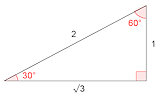# Inverse Trig Calculator

Draw the graph of y = in the range to

Find all angles that have a sine of

#### Common Trig RatiosA self-marking exercise on finding the exact values of sine, cosine and tangent of special angles. Click the image above or the short web address is:

Transum.org/go/?to=commontrigratios

## Inverse Trig Calculator

#### This calculator is designed to find all of the angles for a given trigonometric ratio.

Begin by selecting the trig function and domain using the controls above the graph. Click the Go button to view the graph.

Now enter the value in the box below the graph then click the Find button.

No longer will you need to sketch trig functions in order to demonstrate how to find all solutions to 'simple trigonometric equations in a given interval, including quadratic equations in sin, cos and tan and equations involving multiples of the unknown angle'.

This page is part of the Shine+Write collection, teaching resources ready to be projected onto a whiteboard or screen and written on for whole class discussion. Perfect for interactive whiteboards and large TV screens.For All:

©1997-2021 WWW.TRANSUM.ORG